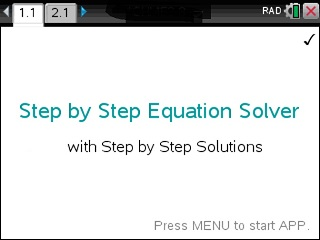# Step by Step Equation Solver

## App Purchase

Enter the last 8 digits of your 27-digit TI-Nspire's Product ID.

Located under 5:Settings → 4:Status → About ID may look like: 1008000007206E210B0BD92F455 HELP. If this was your ID you would only type in BD92F455.

At the end of the PayPal checkout, you will be sent an email containing your app. Read Installation InstructionsPrice:\$49.95

## Description

• Solve any Equations or Systems of Equations Step by Step. Works for any function. Also, performs Step by Step the Quadratic Formula, Complete the Square, Solve Equations via Grouping & Substitution and much more.

## FUNCTIONALITY & MENU ITEMS OF APP :

• Equations
• Step by Step Equation Solver
• Solve any Equation or Inequality
• Solve Equations via Grouping & Substitution
• Complete the Square
• Solve 2x2 system
• Read: PEMDAS - Order of Operation
• Identity Checker
• Newton Method
• Algebra
• Simplify Expression
• Factor
• Expand
• Find Partial Fractions
• Base Conversion: Binary, Hexadecimal, Decimal
• Functions
• Find Slope, Midpoint, Distance given 2 Points
• Find y=mx+b
• All-in-one-Function Explorer
• Evaluate Function
• Find Domain of f(x)
• Find Range of f(x)
• Intersection of 2 Functions
• Composition of 2 Functions f(g(x))
• Complete the Square
• Find [f(x+h)-f(x)]/h
• Find Inverse function
• Newton Method
• Exponents & Logarithms
• Solve any Equation
• Solve using the Rule 72
• Solve Money/Exponential Growth Problems